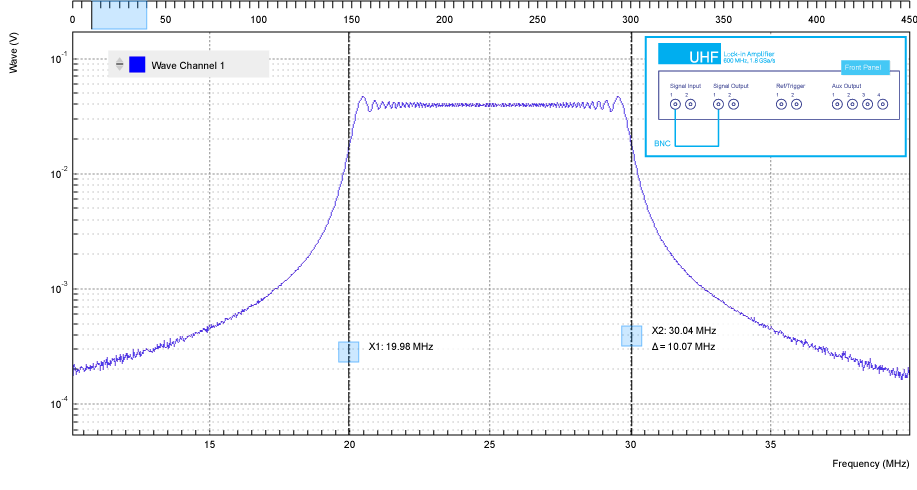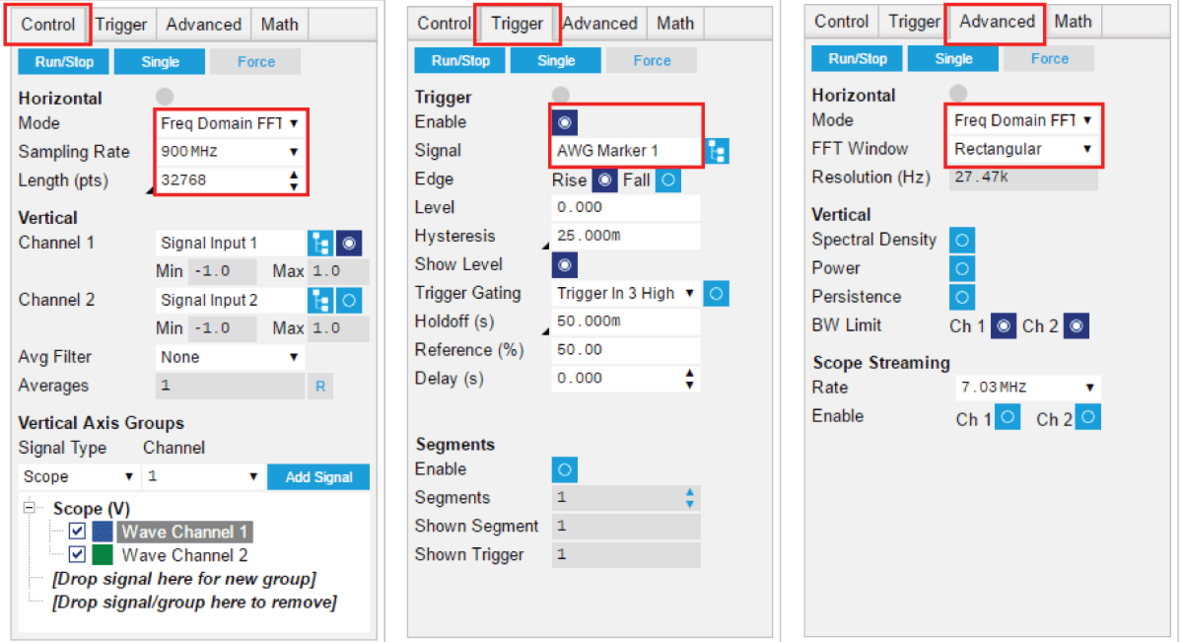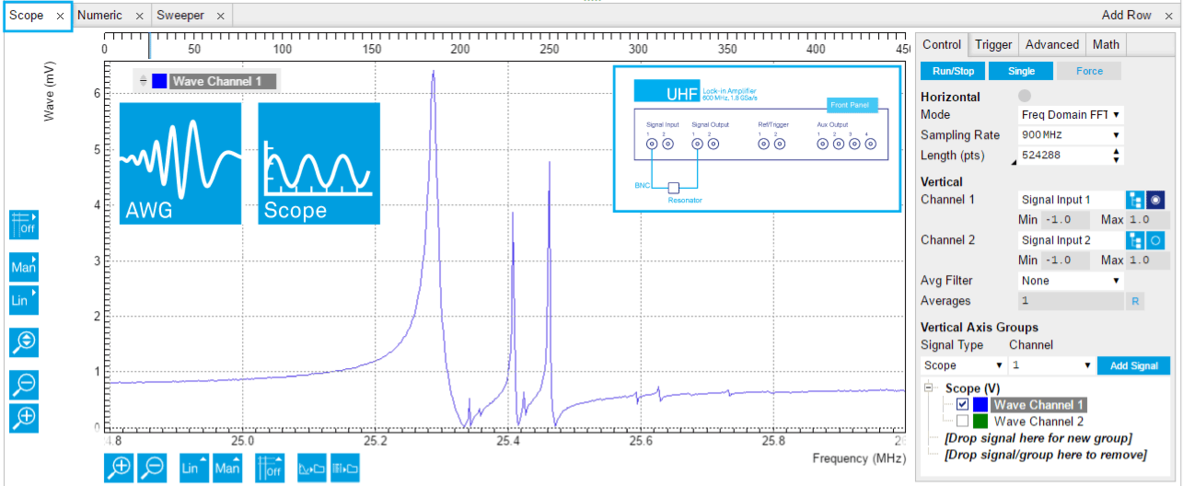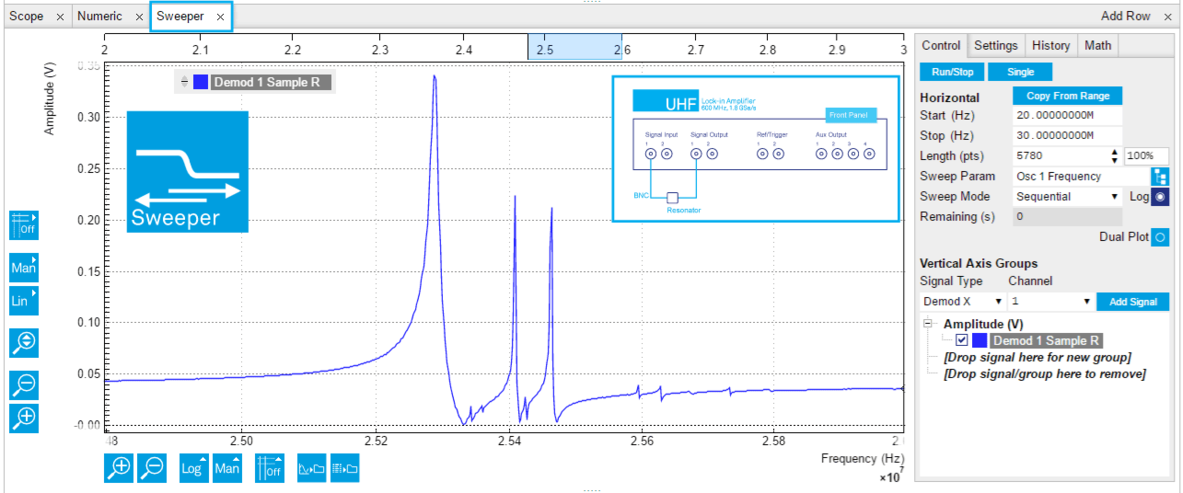# Frequency Response Measurement with Chirps and Leakage-Free FFT

October 28, 2016 by Bruno Küng

## Introduction

This post explains how to measure the frequency response of a component or system with high spectral resolution in a minimum amount of time. Rather than sweeping the frequency and recording each spectral point individually, the approach presented here is based on a fast and continuous change of the frequency while recording the response signal in the time domain simultaneously and then applying a fast Fourier transform (FFT). Proper choice of the sampling rate, the acquisition time and the rate of the frequency change, lead to a spectrum with FFT-based artifacts kept to a minimum.

In this blog post you learn how the scheme can be implemented using the UHFLI Lock-in Amplifier with the UHF-AWG and UHF-DIG options. In the presented example, we win more than a factor of 50 in speed compared to a sweeper measurement. In addition, we provide brief guidelines for deciding whether the method may be advantageous for your application case.

## Measurement Principle and Leakage-Free Operation

The frequency response function is defined as the system response divided by the system drive as a function of frequency. If we choose a drive with a flat spectrum inside the frequency band of interest, we can get the frequency response function from the Fourier transform of the response signal. This is applicable as long as the system is linear.

Discrete-time Fourier transforms such as those employed in digital instruments usually come with an imperfection: spectral leakage. This effect occurs when the measured signal has frequency components that don't fit exactly on the frequency grid (f = 1/T, 2/T, 3/T,...) of the discrete Fourier transform for an acquisition time T. But there's a way to eliminate leakage in our case. The trick is to apply a periodic drive signal with period T. The drive signal, and consequentially the system response signal, will then only contain frequency components at harmonics of 1/T and therefore fit on the FFT grid perfectly.

A good choice for the drive signal is the periodic chirp: a sinusoid with an instantaneous frequency going from fstart to fstop linearly in time, and repeated periodically. The periodic chirp has an almost flat spectrum between fstart and fstop.

## Generating a Chirp

In order to generate such a signal with the UHF-AWG, we store a single period of the chirp as a waveform in the AWG memory and then have the AWG play it repeatedly. The AWG Sequence program below realizes such a signal. It also takes care of three more things:

1. The chirp function begins and ends at 0.
2. The number of samples ns in the waveform is equal to a power of 2.
3. The AWG generates a trigger signal for the UHF-DIG.
// Parameter definition
const f_start = 20e6; // chirp start frequency (Hz)
const f_stop = 30e6; // chirp stop frequency (Hz)
const f_s = 900e6; // AWG ampling frequency (Sa/s)
const t_s = 1/f_s; // AWG sampling period (s)
const A = 1.0; // chirp amplitude (full scale)
const n_s = pow(2,15); // chirp waveform length (samples)
// (condition 2)

// The following calculation adjusts f_stop to ensure
// the chirp waveform starts and ends at 0 (condition 1)
// Chirp rate: frequency span divided by chirp duration
const k = (f_stop-f_start)/(n_s*t_s);
const P = round(n_s*t_s*(f_start + k*n_s*t_s/2));
// New stop frequency (Hz)
const f_stop_adjusted = 2*P/(n_s*t_s) - f_start;

// We generate a chirp waveform using the "chirp" generation function
// and store it in the wave called w_analog:
wave w_analog = A*chirp(n_s, f_start/f_s, f_stop_adjusted/f_s, 0);
// We generate a digital signal to trigger the Scope (condition 3).
// The signal goes from 0 to 1 in the middle of the waveform.
// We store it in the wave called w_marker:
wave w_marker = join(marker(n_s/2, 0b00), marker(n_s/2, 0b01));
// We combine analog and digital data into one waveform called w_chirp:
wave w_chirp = w_analog + w_marker;

// We play the waveform in an infinite "while" loop
while (true){
playWave(w_chirp);
}

Condition 1 ensures that there's no discontinuity of the signal at the point where the start and end of the waveform are stitched together. This is taken care of by a minimal readjustment of fstop ensuring that the chirp sinusoid goes through an integral number of cycles.

Condition 2 is related to the leakage-free Fourier transform. To ensure that the drive signal period matches the acquisition time, the number of samples ns in the AWG waveform must equal the number of samples in a Scope shot. The latter is restricted to powers of 2 to ensure that the acquired data sets can be processed by the fast Fourier transform (FFT) algorithm. Here we picked ns = 215 = pow(2,15) = 32'768.

To play this AWG program, copy the code and paste it into the Sequence Editor in the AWG tab, then click on "Save" to upload it to the instrument. Enable Signal Output 1 and connect it to Signal Input 1, and then run the AWG by clicking on "Single" in the AWG tab. Make sure you reduce the AWG sampling rate to 900 MSa/s. This ensures that AWG and Digitizer can operate smoothly in parallel.

At the end of this post, there are download links to the AWG Sequence program and to a LabOne settings file containing the measurement configuration described in the following.

## Measuring the Chirp

To measure the signal correctly in the Scope tab, we set the Scope Trigger Source to "AWG Marker 1" and enable triggering. Set the "length" parameter to 32'768 samples to fulfill the condition discussed above. Clicking on "Single" will give you a time domain view of the chirp signal. For the frequency domain view, set the Mode to "Frequency Domain FFT", and in the Advanced sub-tab set FFT Window to "Rectangular". Figure 1 shows the frequency domain view of the periodic chirp signal, with Figure 2 highlighting the Scope settings to make in the LabOne user interface. The spectrum of the periodic chirp is almost box-like with edges at fstart = 20 MHz and fstop = 30 MHz. A small ripple towards the edges of the window and a limited roll-off outside are remaining "imperfections" of the chirp function. The UHF-AWG is not limited to periodic chirps: arbitrary excitation signals such as a Schroeder multisine  can be generated as well. Just write the waveform of choice into a CSV file with a tool like MATLAB and import it with the UHF-AWG as explained in the tutorial section of the UHF User Manual.Figure 1: FFT amplitude of the periodic chirp measured with the LabOne Scope. The inset shows the setup used to redirect the UHF-AWG's signal on Signal Output 1 back to the Scope on Signal Input 1.Figure 2: LabOne Scope settings enabling the measurement of a periodic chirp signal generated by the UHF-AWG.

Why use the rectangular FFT window? Window functions such as Hann or Hamming are normally used to counteract spectral leakage. But since there is no leakage here, anything but the rectangular window (i.e., no time-domain windowing) would introduce error.

The importance of the conditions on the periodicity of the signal can easily be understood by playing around with the parameters: choosing a smaller or larger Scope shot length leads to cut-away or comb-like missing parts of the spectrum. Choosing a chirp length incompatible with 2n (e.g., ns = 20'000) will lead to a distorted spectrum affected by leakage.

## Comparing Chirp/FFT to a Sweeper Measurement

Now let's connect a quartz resonator between Signal Output 1 and Signal Input 1 to perform an actual measurement. Figure 3 shows the measurement of the frequency response using the AWG and the digitizer. We used the same AWG program as above but increased the number of samples to ns = 219.Figure 3: Quartz resonator frequency response obtained by applying a periodic chirp drive signal and measuring the response FFT with the LabOne Scope/Digitizer. The measurement took less than 1 s.

Now, we compare the measurement to a swept lock-in measurement between 20 and 30 MHz with the LabOne Sweeper shown in Figure 4. To achieve the same frequency resolution as with the FFT (fs/ns = 900 MHz / 524288 = 1.7 kHz), we need around 6000 points in the 10 MHz sweep range.Figure 4: Quartz resonator frequency response obtained by a lock-in measurement with the LabOne Sweeper. The measurement took about 56 s.

As mentioned in the introduction, the prime argument for using chirp/FFT is speed. In this example, the lock-in sweep took 56 seconds. The chirp/FFT measurement is done in less than 1 second!

Clearly with this single comparison the case is not settled. There is no such thing as a free lunch, and in the case of the chirp/FFT method we pay by having the available drive signal power distributed over a wide frequency range. The method therefore is a trade-off between an advantage, the parallel measurement of many frequencies, and a disadvantage, a reduced signal-to-noise ratio (SNR).

Indeed, chirp/FFT and swept lock-in measurements are equally efficient in terms of signal processing - they yield roughly the same SNR in the same effective measurement time! But in many practical situations, it's the overhead (instrument communication, filter settling, device ring-up) rather than the effective measurement time which really limits the sweeper measurement speed. In our example, instrument communication limited the sweeper speed to about 10 ms per point, which is why the chirp/FFT method was much faster.

The frequency resolution of the chirp/FFT measurement is equal to fs divided by the number of points ns. Both the UHF-AWG and the UHF-DIG support up to 128 MSa memory for pushing the resolution to very high levels by increasing the number of points. If the system under test allows a measurement at reduced frequency, reducing the sampling rate fs of the AWG and Digitizer is another way to increase frequency resolution. High frequency resolution is a key requirement for testing high-Q resonators: since there should be at least a couple of points inside the resonance of such a device, the maximum number of samples directly relates to the accessible Q factors with a chirp/FFT measurement.

Note that the frequency resolution does not improve when reducing the frequency span fstop-fstart of the chirp! Nonetheless it's better to choose a narrow frequency range if possible as this leads to a higher SNR due to more drive power spectral density. Also the chirp rate (fstop-fstart)/T is only indirectly affecting the frequency resolution via the measurement time T.

The table below summarizes the free parameters and how the measurement is affected when increasing them.

 Parameter Effect Trade-off frequency span fstop-fstart increases measurement range lower SNR waveform length ns increases frequency resolution longer waveform upload time sampling rate fs increases maximum frequency poorer frequency resolution number of Scope averages increases SNR longer measurement time

## Summary

The chirp/FFT measurement is a fast and powerful method for measuring the frequency response of linear systems. Speeding up the frequency response measurement matters, e.g. for imaging applications such as laser scanning vibrometry, due to the large number of spectra that need to be acquired. It's also welcome for the characterization of high-Q resonators (MEMS) where the sweep speed is limited by the ring-up time of the device under test.

From the discussion above, we can distill a couple of conditions indicating that the chirp/FFT method is likely to be faster than a sweeper measurement. Take them as qualitative guidelines:

• The sweeper speed is limited by the instrument communication time.
• The sweeper speed is limited by the device settling time (e.g., resonator ring-up time).
• The measurement signal is strong and noise is not a big issue.

The chirp/FFT is not suited to all cases, though. The following criteria indicate that the chirp/FFT method is unsuited, or at least brings no improvement, compared to a swept lock-in measurement:

• The sweeper speed is limited by noise and averaging time.
• The required frequency resolution exceeds the limits set by the UHF-AWG and UHF-DIG memory.
• The device under test is nonlinear.